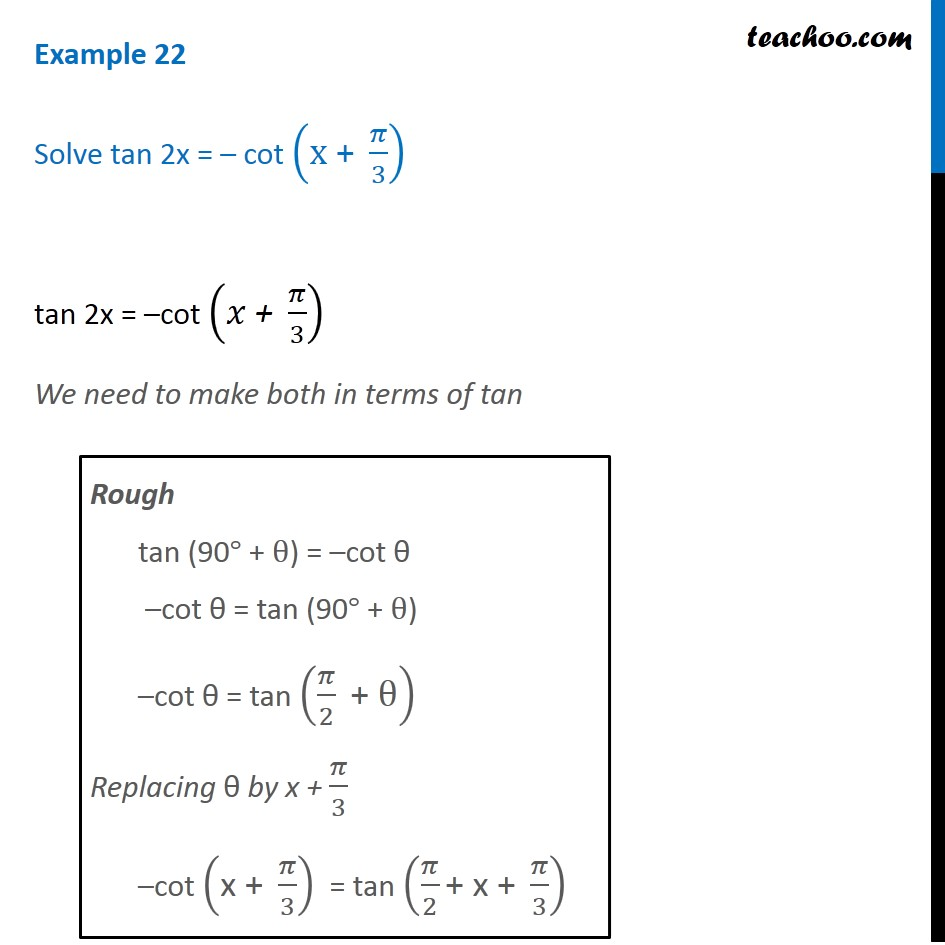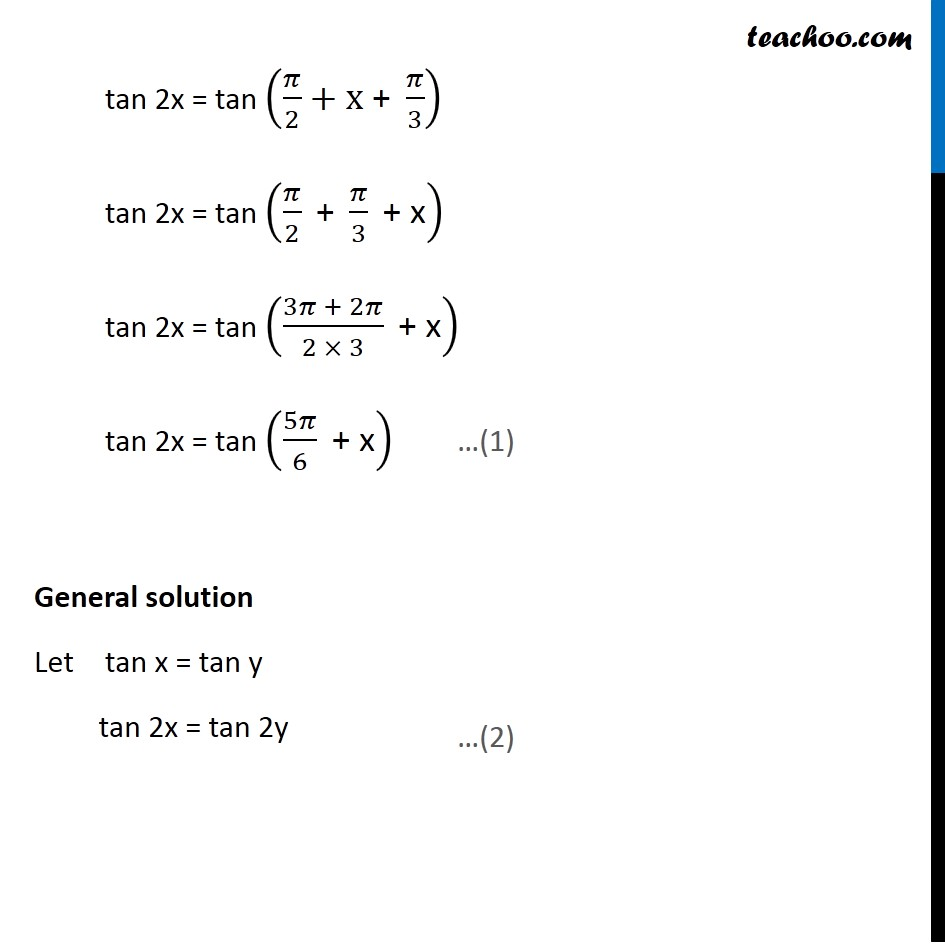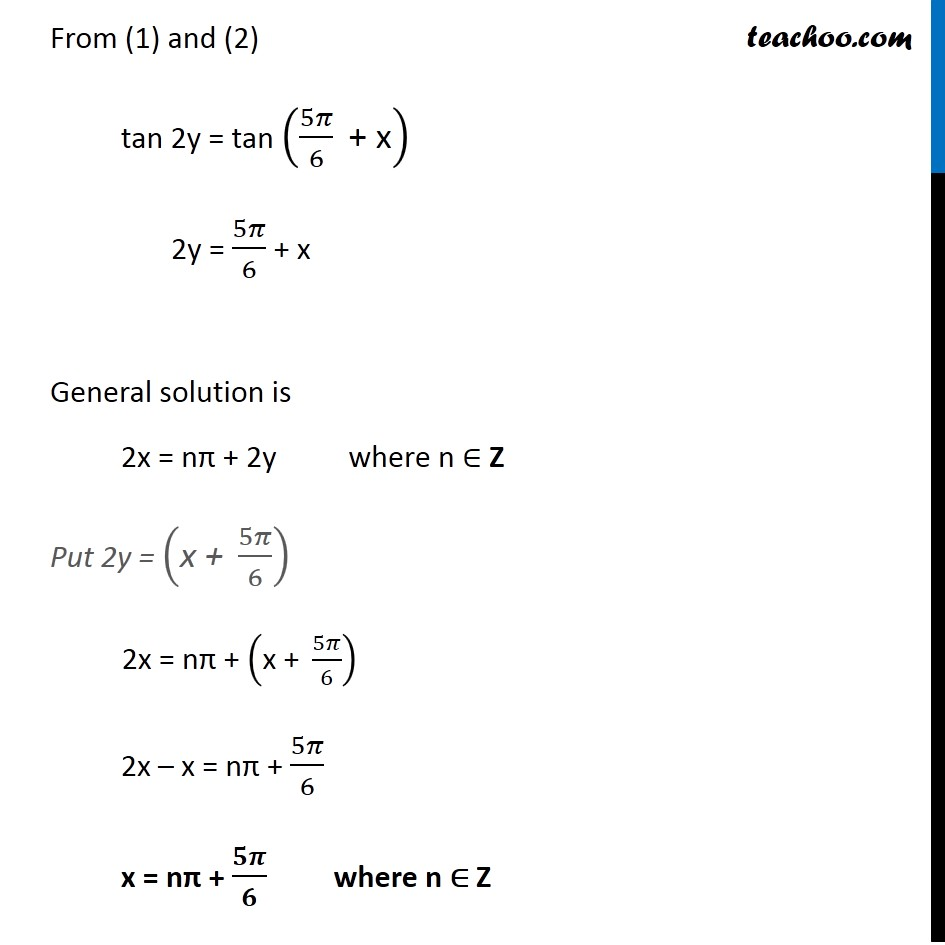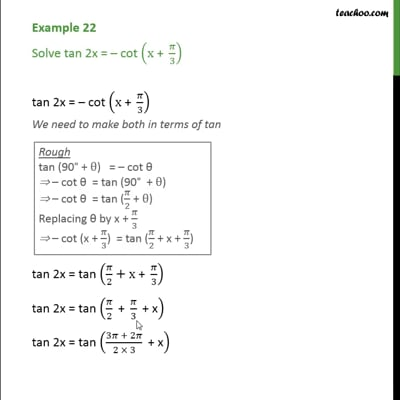Finding General Solutions

Chapter 3 Class 11 Trigonometric Functions
Concept wiseThis video is only available for Teachoo black users

Introducing your new favourite teacher - Teachoo Black, at only ₹83 per month

### Transcript

Example 22 Solve tan 2x = – cot (x" + " 𝜋/3) tan 2x = –cot (𝑥" + " 𝜋/3) We need to make both in terms of tan Rough tan (90° + θ) = –cot θ –cot θ = tan (90° + θ) –cot θ = tan (𝜋/2 " + θ" ) Replacing θ by x + 𝜋/3 –cot ("x + " 𝜋/3) = tan (𝜋/2 "+ x +" 𝜋/3) tan 2x = tan (𝜋/2+x" + " 𝜋/3) tan 2x = tan (𝜋/2 " + " 𝜋/3 " + x" ) tan 2x = tan ((3𝜋 + 2𝜋)/(2 × 3) " + x" ) tan 2x = tan (5𝜋/6 " + x" ) General solution Let tan x = tan y tan 2x = tan 2y From (1) and (2) tan 2y = tan (5𝜋/6 " + x" ) 2y = 5𝜋/6 + x General solution is 2x = nπ + 2y where n ∈ Z Put 2y = ("x + " 5𝜋/6) 2x = nπ + ("x + " 5𝜋/6) 2x – x = nπ + 5𝜋/6 x = nπ + 𝟓𝝅/𝟔 where n ∈ Z